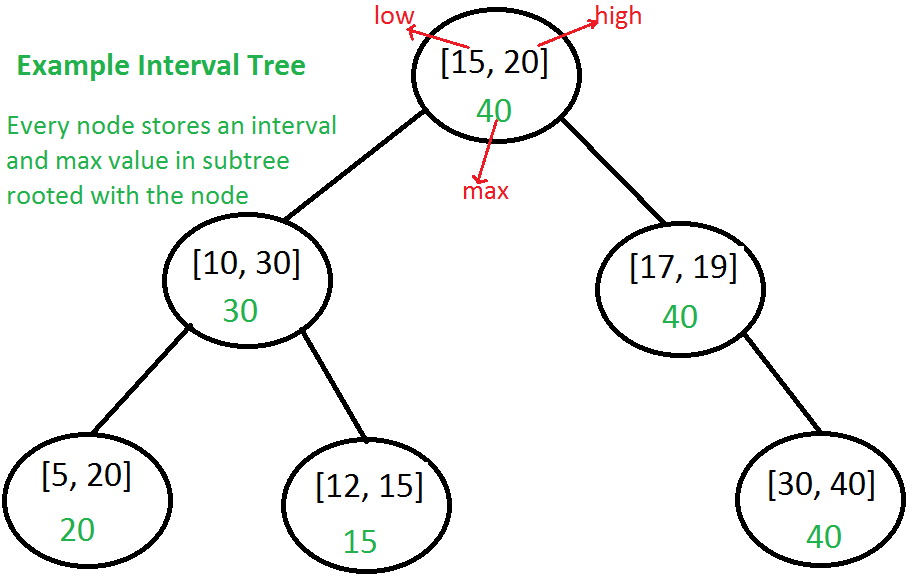### Interval Tree

Consider a situation where we have a set of intervals and we need following operations to be implemented efficiently.

1. Add an interval
2. Remove an interval
3. Given an interval x, find if x overlaps with any of the existing intervals.

Interval Tree: The idea is to augment a self-balancing Binary Search Tree (BST) like Red Black Tree, AVL Tree, etc to maintain set of intervals so that all operations can be done in O(Logn) time.

Every node of Interval Tree stores following information.

1. An interval which is represented as a pair [low, high]
2. Maximum high value in subtree rooted with this node.

The low value of an interval is used as key to maintain order in BST. The insert and delete operations are same as insert and delete in self-balancing BST used.The main operation is to search for an overlapping interval. Following is algorithm for searching an overlapping interval x in an Interval tree rooted with root.